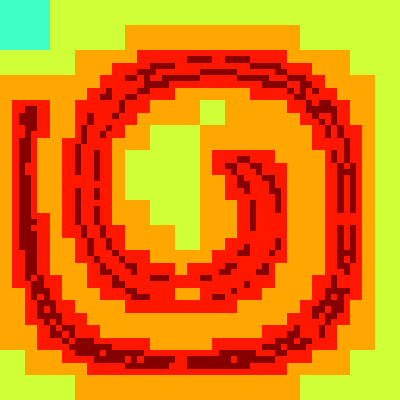# Time-reversed VOF advection in a vortex

This classical test advects and stretches an initially circular interface in a non-divergent vortical flow. The flow reverts in time and the interface should come back to its original position. The difference between the initial and final shapes is a measure of the errors accumulated during advection.

This also checks that a constant “VOF concentration” remains constant when it is advected with the VOF tracer.

We will need the advection solver combined with the VOF advection scheme.

#include "advection.h"
#include "vof.h"

The volume fraction is stored in scalar field f which is listed as an interface for the VOF solver. We do not advect any tracer with the default (diffusive) advection scheme of the advection solver.

scalar f[], cf[];
scalar * interfaces = {f}, * tracers = NULL;
int MAXLEVEL;

We center the unit box on the origin and set a maximum timestep of 0.1

int main()
{
origin (-0.5, -0.5);
DT = .1[0,1];

The scalar field cf is a “vof concentration” associated with phase f.

  f.tracers = {cf};

We then run the simulation for different levels of refinement.

  for (MAXLEVEL = 5; MAXLEVEL <= 7; MAXLEVEL++) {
init_grid (1 << MAXLEVEL);
run();
}
}

The initial interface is a circle of radius 0.2 centered on (-0.2,-0.236338) (for historical reasons). We use the levelset function circle() to define this interface.

The period of the stretching cycle is set to 15, which will lead to strong stretching. Milder conditions can be obtained by decreasing it.

#define circle(x,y) (sq(0.2) - (sq(x + 0.2) + sq(y + .236338)))
const double T = 15.;

We define the levelset function \phi on each vertex of the grid and compute the corresponding volume fraction field.

event init (i = 0)
{
fraction (f, circle(x,y));
foreach()
cf[] = f[];
}

event velocity (i++) {

This event defines the velocity field.

On trees we first adapt the grid so that the estimated error on the volume fraction is smaller than 5\times 10^{-3}. We limit the resolution at MAXLEVEL and we only refine the volume fraction field f and associated tracer cf.

#if TREE
adapt_wavelet ({f}, (double[]){5e-3}, MAXLEVEL, list = {f, cf});
#endif

The velocity field is defined through a streamfunction \psi, defined on the vertices of the grid.

  vertex scalar psi[];
double a = 1.5, k = pi;
foreach_vertex()
psi[] = - a*sin(2.*pi*t/T)*sin(k*(x + 0.5))*sin(k*(y + 0.5))/pi;

We can then differentiate the streamfunction to get the velocity components. This guarantees that the velocity field is exactly non-divergent.

  trash ({u});
struct { double x, y; } f = {-1.,1.};
foreach_face()
u.x[] = f.x*(psi[0,1] - psi[])/Delta;
}

At the start and end of the simulation we check the sum, min and max values of the volume fraction field. The sum must be constant to within machine precision and the volume fraction should be bounded by zero and one.

event logfile (t = {0,T}) {
stats s = statsf (f);

We compute the minimum and maximum concentration. They should both be equal to one.

  stats sc = statsf (cf);
double cmin = HUGE, cmax = 0.;
foreach (reduction(min:cmin) reduction(max:cmax))
if (f[] > 1e-12) { // round-off errors are a problem
double c = cf[]/f[];
if (c < cmin) cmin = c;
if (c > cmax) cmax = c;
}
fprintf (stderr, "# t\t\tf.sum\t\tf.min\t\tf.max\n");
fprintf (stderr, "# %f %.12f %.f %g\n", t, s.sum, s.min, s.max);
fprintf (stderr, "# t\t\tcf.sum\t\tc.min - 1\tc.max - 1\n");
fprintf (stderr, "# %f %.12f %.11f %.11f\n",
t, sc.sum, fabs(cmin - 1.), fabs(cmax - 1.));
}

To compute the error, we reinitialise field e at the end of the simulation with the initial shape and compute the difference with the final shape. We output the norms as functions of the maximum resolution N.

event field (t = T) {
scalar e[];
fraction (e, circle(x,y));
foreach()
e[] -= f[];
norm n = normf (e);
fprintf (stderr, "%d %g %g %g\n", N, n.avg, n.rms, n.max);
}

We also output the shape of the reconstructed interface at regular intervals (but only on the finest grid considered).

event shape (t += T/4.) {
if (N == 128)
output_facets (f);
}

If we are using adaptivity, we also output the levels of refinement at maximum stretching.

#if TREE
event levels (t = T/2) {
if (N == 128) {
scalar l[];
foreach()
l[] = level;
output_ppm (l, file = "levels.png", n = 400, min = 0, max = 7);
}
}
#endif

#if 0
event movie (i += 10)
{
scalar l[];
foreach()
l[] = level;
output_ppm (l, 1 << MAXLEVEL, file = "level.mp4");
}
#endif

## Results

We use gnuplot to compute the convergence rate of the error norms with and without adaptation. The convergence rates are comparable.

ftitle(a,b) = sprintf("%.0f/x^{%4.2f}", exp(a), -b)

f(x)=a+b*x
fit f(x) 'log' u (log($1)):(log($4)) via a,b
f2(x)=a2+b2*x
fit f2(x) 'log' u (log($1)):(log($2)) via a2,b2

fc(x)=ac+bc*x
fit fc(x) 'clog' u (log($1)):(log($4)) via ac,bc
fc2(x)=ac2+bc2*x
fit fc2(x) 'clog' u (log($1)):(log($2)) via ac2,bc2

set xlabel 'Maximum resolution'
set ylabel 'Maximum error'
set key bottom left
set logscale
set xrange [16:256]
set xtics 16,2,256
set grid ytics
set cbrange [1:1]
plot 'log' u 1:4 t 'max (adaptive)', exp(f(log(x))) t ftitle(a,b), \
'clog' u 1:4 t 'max (constant)', exp(fc(log(x))) t ftitle(ac,bc), \
'log' u 1:2 t 'norm1 (adaptive)', exp(f2(log(x))) t ftitle(a2,b2), \
'clog' u 1:2 t 'norm1 (constant)', exp(fc2(log(x))) t ftitle(ac2,bc2)Convergence rates for constant- and adaptive grids. (script)

The shapes of the interface at t=0, t=T/4, t=T/2, t=3T/4 and t=T are displayed below for both sets of simulations (constant and adaptive), for N=128. The shapes for t=T/4 should be identical to those for t=3T/4 and similarly for t=0 and t=T (for which we measure the error). Note that the errors for t=3T/4 seem to be much larger than those for t=T.

reset
set size ratio -1
plot [-0.5:0.5][-0.5:0.5]'out' w l t "adaptive", 'cout' w l t "constant"Shapes of the interface for t=0, t=T/4, t=T/2, t=3T/4 and t=T for two sets of simulations. (script)Refinement levels for t=T/2 and N=128.